# The Dragon Curve

Let me tell you about a beautiful, fractal curve, the Dragon Curve. Download my new dragon program from the File Exchange and follow along.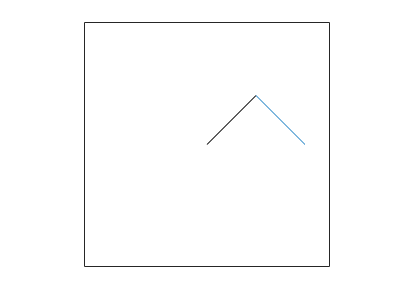### Contents

#### Folding paper

Start with a long, narrow strip of paper, like the one in the following photograph. Fold it in half once, end over end. Then fold it a second time, being careful to fold in the same direction, right over left or left over right, as the first. Then a third fold, and a fourth. Unfold the strip so that all the folds form the right angles pictured. You will have created a Dragon Curve of degree four.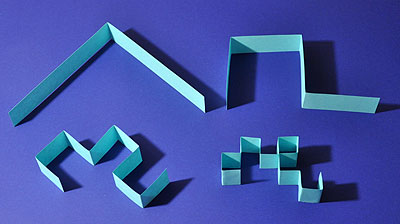Image credit: http://www.cutoutfoldup.com/216-dragon-curve.php

How many times do you imagine you could fold the paper before it is too thick to fold again? Probably five, maybe six.

#### Signature

The degree one curve has one right angle; let's denote that by R. The degree two curve has two right angles, followed by a left; that's RRL. The degree three signature is RRLRRLL.

Can you read off the signature of degree four from the photo? Do you see the pattern? Probably not yet.

The number of segments between folds doubles with each increase in degree. Here's code to display the signatures of degrees one through six. That's all I can display in one column of this blog post.

The key operation in the code is LR-fliplr(s). That flips the string s end to end and replaces all the R's by L's and the L's by R's.

    s = 'R';
disp(s)
LR = 'L'+'R';
for n = 2:6
s = [s 'R' LR-fliplr(s)];
disp(s)
end

R
RRL
RRLRRLL
RRLRRLLRRRLLRLL
RRLRRLLRRRLLRLLRRRLRRLLLRRLLRLL
RRLRRLLRRRLLRLLRRRLRRLLLRRLLRLLRRRLRRLLRRRLLRLLLRRLRRLLLRRLLRLL


#### Folds

The following figures are all from my new MATLAB program, dragon. The computations are based on a growing and shrinking vector z of points in the complex plane.

  z = [0 1]

This isn't complex yet, but it soon will be. When we plot z, we get a single black line, representing an unfolded strip of paper.Let's fold the paper once. This is done with the help of the complex number

  w = (1+i)/2

In polar form, $w$ is $e^{i \pi/4}/\sqrt{2}$. So complex multiplication by $w$ rotates by $45^\circ$ and scales by $1/\sqrt{2}$.

In MATLAB, w' is the complex conjugate of w. This is used in the "fold" operation for any vector z.

  zleft = w*z
zright = 1 - w'*fliplr(z)
z = [zleft zright]

Folding turns [0 1] into

  [0 w w 1]

When we plot any vector, we break it in half, then plot the left half in black and the right half in blue.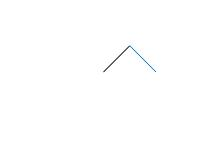The black line has been rotated $45^\circ$ to the left and a blue line, rotated $45^\circ$ to the right, appended.

Fold it again.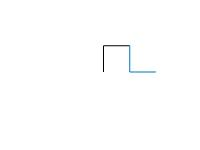The black line becomes a black right angle and the blue line becomes a blue "left angle".

Two more folds produce a degree four Dragon Curve.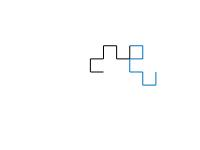A total of eight folds gives degree eight.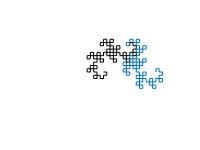And after a total of eighteen folds, we have our dragon.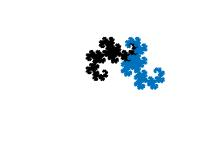We could keep on folding, but we now have $2^{18}$ short line segments and have reached the limits of screen resolution. More folds do not change any more pixels.

#### Rotations

Let's do a different kind of rotation. Rotate the entire dragon by $90^\circ$, superimpose it on the original, and plot the four halves with four colors.Do it twice more.#### Translates

We could now do translations in all four compass directions and superimpose all the dragons, but I won't show that. It turns out that as both the degrees and the translates go to infinity, the curves, which are intricately intertwined, never intersect, and completely cover and tile the complex plane.

#### Pendant

I found this at an on-line shopping center named Etsy, in the "Fractal and Geeky Jewelry" section. It's from an outfit named DragonNerd in Winnipeg. Count the number of bends to determine the degree.

The last time I blogged about jewelry was in my piece about the Pentium Affair.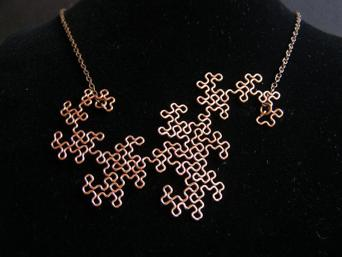Image credit: http://www.etsy.com/shop/DragonNerd

#### Dragon

All the figures above are from my new program, dragon, available from MATLAB Central and also included in Cleve's Laboratory.

dragon provides four pushbuttons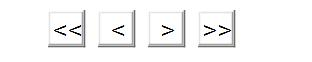These buttons

• Unwind the rotations
• Reduce the degree
• Increase the degree
• Superimpose rotations

#### Videos

I highly recommend two videos from Numberphile. In one, Don Knuth tells about a wrong turn he made in the Dragon Curve ceramic displayed in his home, https://www.youtube.com/watch?v=v678Em6qyzk.

In the other, the popular British author, Rob Eastaway, describes the appearance of the Curve in Michael Crichton's Jurassic Park, https://www.youtube.com/watch?v=wCyC-K_PnRY.

Published with MATLAB® R2018a

|